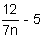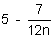# Challenge Exercises for Pre-Algebra

Short Answer Directions: Read each question. Click once in an ANSWER BOX and type in your answer. After you click ENTER, a message will appear in the RESULTS BOX to indicate whether your answer is correct or incorrect. To start over, click CLEAR.

Multiple Choice Directions: Select your answer by clicking on its button. Feedback to your answer is provided in the RESULTS BOX. If you make a mistake, choose a different button.

 1 An agent charges \$150 per gig to book a rock band, plus \$75 per month for travel expenses. What was his monthly fee if he booked 6 gigs for the band last month? ANSWER BOX: \$   RESULTS BOX:
 2 A gardener charges \$8 per square foot to lay sod. If a square garden is 7 feet along each side, how much will he charge to lay sod on it? ANSWER BOX: \$   RESULTS BOX:
 3 Six people in a club will share the expenses of a party that costs \$240. How much will Katie pay for her share of the party if the club owes her \$8?  ANSWER BOX: \$   RESULTS BOX:
 4 Evaluate the following expression:ANSWER BOX:   RESULTS BOX:
5.  Jesse spends \$5 a day on lunch. Which algebraic expression correctly represents the amount of money he will spend on lunch in x days?

 x - 5 5x 5 + x None of the above.
 RESULTS BOX:
6.  Which algebraic expression correctly represents this phrase?
The quotient of twelve and seven times a number, decreased by five.None of the above.
 RESULTS BOX:
 7. Which algebraic equation correctly represents this sentence? A number increased by eight is nineteen. 19 - y = 819 + y = 8y + 8 = 19None of the above. RESULTS BOX:
 8. Which algebraic equation correctly represents this sentence? Twenty-five is three times a number, decreased by eight. 25 = 3w - 83 = 25w - 83 = 25w + 8None of the above. RESULTS BOX:
 9. Which sentence correctly represents this algebraic equation? 15 = 16y - 3 Fifteen is sixteen times the difference of three and a number.Fifteen is sixteen times the difference of a number and three.Fifteen times three is sixteen times a number.None of the above. RESULTS BOX:
 10. Which sentence correctly represents the algebraic equation below?The quotient of five and three times a number is thirty.The quotient of three times a number and five is thirty.Thirty is the product of three times a number and five.None of the above. RESULTS BOX: